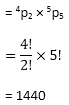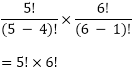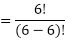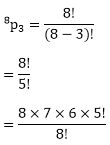×#### Thank you for registering.

One of our academic counsellors will contact you within 1 working day.

Click to Chat

1800-1023-196

+91-120-4616500

CART 0

• 0

MY CART (5)

Use Coupon: CART20 and get 20% off on all online Study Material

ITEM
DETAILS
MRP
DISCOUNT
FINAL PRICE
Total Price: Rs.

There are no items in this cart.
Continue Shopping• Complete JEE Main/Advanced Course and Test Series
• OFFERED PRICE: Rs. 15,900
• View Details

```Chapter 16: Permutations – Exercise 16.4

Permutations – Exercise – 16.4 – Q.1

There are 4 vowels and 3 consonants in the word 'FAILURE'

We have to arrange 7 letters in a row such that consonants occupy odd places. There are 4 odd places (1, 3, 5, 7). There consonants can be arranged in these 4 odd places in 4P3 ways.

Remaining 3 even places (2, 4, 6) are to be occupied by the 4 vowels. This can be done in 4P3 ways.

Hence, the total number of words in which consonants occupy odd places = 4P3 × 4P3= 4 × x 3 × 2 × 1 × 4 × 3 × 2 × 1

= 24 × 24

= 576.

Permutations – Exercise – 16.4 – Q.2

There are 7 letters in the word 'STRANGE', including 2 vowels (A, E) and 5 consonants (S, T, R, N, G).

(i) Considering 2 vowels as one letter, we have 6 letters which can be arranged in 6p6 = 6! ways A,E can be put together in 2! ways.

Hence, required number of words

= 6i × 2!

= 6 × 5 × 4 × 3 × 2 × 1 × 2

= 720 × 2

= 1440.

(ii) The total number of words formed by using all the letters of the words 'STRANGE' Is 7p7 = 7!

= 7 × 6 × 5 × 4 × 3 × 2 × 1

= 5040.

So, the total number of words in which vowels are never together

= Total number of words – Number of words in which vowels are always together

= 5040 - 1440

= 3600

(iii) There are 7 letters in the word 'STRANGE'. out of these letters 'A' and 'E' are the vowels. There are 4 odd places in the word 'STRANGE'. The two vowels can be arranged in 4p2 ways. The remaining 5 consonants can be arranged among themselves in 5p5 ways.

The total number of arrangementsPermutations – Exercise – 16.4 – Q.3

There are 6 letters in the word 'SUNDAY'. The total number of words formed with these 6 letters is the number of arrangements of 6 items, taken all at a time, which is equal

to 6p6 = 6! = 6 × 5 × 4 × 3 × 2 × 1 = 720.

If we fix up D in the beginning, then the remaining 5 letters can be arranged in 5P5 = 5! ways.

so, the total number of words which begin with D = 5!

= 5 × 4 × 3 × 2 × 1 = 120.

Permutations – Exercise – 16.4 – Q.4

There are 4 vowels and 4 consonants in the word 'ORIENTAL'. We have to arrange 8 leeters in a row such that vowels occupy odd places. There are 4 odd places (1, 3, 5, 7). Four vowels can be arranged in these 4 odd places in 4! ways. Remaining 4 even places (2, 4, 6, 8) are to be occupied by the 4 consonants.

This can be done in 4! ways.

Hence, the total number of words in which vowels occupy odd places = 4! × 4!

= 4 × 3 × 2 × 1 × 4 × 3 × 2 × 1 = 576.

Permutations – Exercise – 16.4 – Q.5

There are 6 letters in the word 'SUNDAY'. The total number of words formed with these 6 letters is the number of arrangements of 6 items, taken all at a time, which is equal to 6p6 = 6!

= 6 × 5 × 4 × 3 × 2 × 1 = 720.

If we fix up N in the begining, then the remaining 5 letters can be arranged in 5p5 = 5! ways so, the total number of words which begin which N = 5!

= 5 × 4 × 3 × 2 × 1

= 120

if we fix up N in the begining and Y at the end, then the remaining 4 letters can be arranged in 4p4 = 4! ways.

So, the total number of words which begin with N and end with Y = 4! = 4 × 3 × 2 × 1 = 24.

Permutations – Exercise – 16.4 – Q.6

There are 10 letters in the word 'GANESHPUR1'. The total number of words formed is equal to 10p10 = 10!

(i) If we fix up G in the begining, then the remaining 9 letters can be arranged in 9p9 = 9! ways

(ii) If we fix up P in the begining and I at the end, begining 8 letters can be arranged in 8p8 = 8!.

(iii) There are 4 vowels and 6 consonants in the word 'GANESHPURI'.

Considering 4 vowels as one letter,

We have 7 letters which can be arranged in 7p7 = 7! ways.

A,E,U,I can be put together in 4! ways.

Hence, required number of words = 7! × 4!.

(iv) We have to arrange 10 letters in a row such that vowels occupy even places. There are 5 even places (2, 4, 6, 8, 10), 4 vowels can be arranged in these 5 even places in 5p4 ways.

Remaining 5 odd places (1, 3, 5, 7, 9) are to be occupied by the 6 consonants.

This can be done in 6C5 ways.

Hence, the total number of words in which vowels occupy even places = 5p4 × 6P5Permutations – Exercise – 16.4 – Q.7

(i) There are 6 letters in the word 'VOWELS'. The total number of words formed with these 6 letters is the number of arrangements of 6 items, taken all at a time, which is equal to

6p6 = 6! = 6 × 5 × 4 × 3 × 2 × 1 = 720

(ii) If we fix up E in the begining then the remaining 5 letters can be arranged in

5p5 = 5! = 5 × 4 × 3 × 2 × 1 = 120 ways

(iii) If we fix up 0 in the begining and L at the end, the remaining 4 letters can be arranged in

4p4 = 4! = 4 × 3 × 2 × 1 = 24.

(iv) There are 2 vowels and 4 consonants in the word 'VOWELS'. Considering 2 vowels as one letter, we have letters which can be arranged in 5p5 = 5! ways.

O, E can be put together in 2! ways.

Hence, required number of

words = 5! × 2!

= 5 × 4 × 3 × 2 × 1 × 2 × 1

= 120 × 2

= 240

(v) There are 2 vowels and 4 consonants in the word 'VOWELS'.

Considering 4 consonants as one letter, we have 3 letters which can be arranged in 3p3 = 3! ways. U, W, L, S can be put together in 4! ways.

Hence, required number of words in which all consonants come together = 3! × 4!

= 3 × 2 × 4 × 3 × 2

= 144.

Permutations – Exercise – 16.4 – Q.8

We have to arrange 7 letters in a row such that vowels occupy even places.

There are 3 even places (2, 4, 6). Three vowels can be arranged in these 3 even places in 3! ways.

Remaining 4 odd places (1, 3, 5, 7) are to be occupied by the 4 consonants. This can be done in 4! ways.

Hence, the total number of words in which vowels occupy even places = 3! × 4!

= 3 × 2 × 4 × 3 × 2 = 144

Permutations – Exercise – 16.4 – Q.9

Let two husbands A, B be selected out of seven males in = 7C2 ways. excluding

their wives, we have to select two ladies C,D out of remaining 5 wives is = 5C2 ways.

Thus, number of ways of selecting the players for mixed double is = 7C2 × 5C2

= 21 × 10

= 210

Now, suppose A chooses C as partner (B will automatically go to D) or A chooses 0 as partner (B will automatically go to C) Thus we have, 4 other ways for teams.

Required number of ways = 210 × 4 = 840

Permutations – Exercise – 16.4 – Q.10

m men can be seated in a row in mpm = m! ways.

Now, in the (m + 1) gaps n women can be arranged in m+1pn ways.

Hence, the number of ways in which no two women sit togetherHence, proved

Permutations – Exercise – 16.4 – Q.11

(i) MONDAY has 6 letters with no repetitions, so

Number of words using 4 letters at a time with no repetitions = 6p4

= 6!/2!

= 360

(ii) Number of words using all 6 letters at a time with no repetitions = 6p6= 6 × 5 × 4 × 3 × 2 × 1

= 720

(iii) Number of words using all 6 letters, starting with vowels

= 2,5p5

= 2 × 5 × 4 × 3 × 2 × 1

= 240

Permutations – Exercise – 16.4 – Q.12

There are 8 letters in the word 'ORIENTAL'. The total number of three letter words is the number of arrangements of 8 items, taken 3 at a time, which is equal to= 336.

Hence, the total number three letter words are 336.

```### Course Features

• 728 Video Lectures
• Revision Notes
• Previous Year Papers
• Mind Map
• Study Planner
• NCERT Solutions
• Discussion Forum
• Test paper with Video Solution